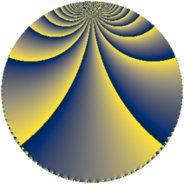# Properties

 Label 252.4.baLevel $252$ Weight $4$ Character orbit 252.ba Rep. character $\chi_{252}(155,\cdot)$ Character field $\Q(\zeta_{6})$ Dimension $216$ Sturm bound $192$

# Learn more

## Defining parameters

 Level: $$N$$ $$=$$ $$252 = 2^{2} \cdot 3^{2} \cdot 7$$ Weight: $$k$$ $$=$$ $$4$$ Character orbit: $$[\chi]$$ $$=$$ 252.ba (of order $$6$$ and degree $$2$$) Character conductor: $$\operatorname{cond}(\chi)$$ $$=$$ $$36$$ Character field: $$\Q(\zeta_{6})$$ Sturm bound: $$192$$

## Dimensions

The following table gives the dimensions of various subspaces of $$M_{4}(252, [\chi])$$.

Total New Old
Modular forms 296 216 80
Cusp forms 280 216 64
Eisenstein series 16 0 16

## Trace form

 $$216q - 30q^{6} - 20q^{9} + O(q^{10})$$ $$216q - 30q^{6} - 20q^{9} - 156q^{12} + 290q^{18} + 462q^{20} + 382q^{24} + 2700q^{25} - 716q^{30} - 690q^{32} + 892q^{33} + 396q^{34} - 412q^{36} + 180q^{40} + 300q^{41} + 140q^{42} + 984q^{45} - 72q^{46} + 1652q^{48} + 5292q^{49} + 3198q^{50} + 918q^{52} + 1826q^{54} - 172q^{57} + 594q^{58} + 2036q^{60} - 828q^{64} - 696q^{65} - 7446q^{66} - 4602q^{68} + 744q^{69} - 4870q^{72} + 1656q^{73} - 9072q^{74} + 1116q^{76} - 4268q^{78} - 1996q^{81} - 1404q^{82} - 490q^{84} + 4170q^{86} - 2088q^{88} + 15320q^{90} + 9462q^{92} - 672q^{93} - 306q^{94} + 3280q^{96} - 36q^{97} + O(q^{100})$$

## Decomposition of $$S_{4}^{\mathrm{new}}(252, [\chi])$$ into newform subspaces

The newforms in this space have not yet been added to the LMFDB.

## Decomposition of $$S_{4}^{\mathrm{old}}(252, [\chi])$$ into lower level spaces

$$S_{4}^{\mathrm{old}}(252, [\chi]) \cong$$ $$S_{4}^{\mathrm{new}}(36, [\chi])$$$$^{\oplus 2}$$